## Example Questions

2 Next →

### Example Question #381 : Arithmetic

Evaluate: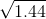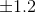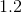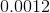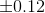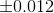Explanation:

The square root of a number returns a positive and negative number that multiplies by itself to obtain the number inside the square root.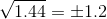### Example Question #382 : Arithmetic

Without using a calculator, solve for x: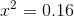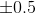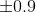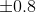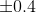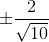Explanation:

Simplifying the given equation gives us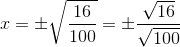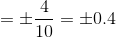Therefore, the correct answer is.

### Example Question #383 : Arithmetic

If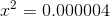and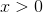, what is the value of?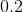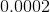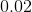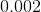Explanation:

We are given the equation. To determine the value of, take the square root of both sides.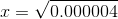.

To calculate this value, take note of the following pattern: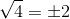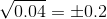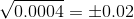Each succeeding radicand is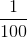of the previous radicand, and the value of each succeeding square root is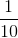of the previous value. Continuing the pattern: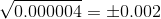. However, we are also given the condition that; hence, we eliminate the extraneous solution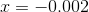and conclude that the only valid solution ofis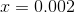.

2 Next →